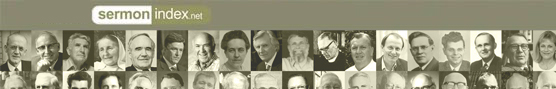Promoting Genuine Biblical Revival.
Sermon Podcast | Audio | VideoSermonIndex.net : Christian Books : PART SECOND.--STORIES OF THE EARLY CHURCH.

Hurlbuts Bible Lessons by Rev. Jesse Lyman Hurlbut

### PART SECOND.--STORIES OF THE EARLY CHURCH.

Lesson XXXV. The First Days.

(Tell Story 1 in Part Seventh.)

=1.= Where did the followers of Chr[=i]st meet after J[=e]´[s+]us went away to heaven? =In J[+e]-r[u:]´s[+a]-l[)e]m.=

=2.= What day came ten days after J[=e]´[s+]us left the earth? =The Day of P[)e]n´te-c[)o]st.=

=3.= What seemed to fall from heaven on all the followers of Chr[=i]st on the day of P[)e]n´te-c[)o]st? =Tongues of fire.=

=4.= What power came upon them all? =Power from God.=

=5.= What did they all begin to speak? =The wonderful words of God.=

=6.= Who preached to the people on that day? =P[=e]´t[e]r.=

=7.= What new name was given to P[=e]´t[e]r and the other eleven disciples of Chr[=i]st? =They were called apostles.=

=8.= What did the apostle P[=e]´t[e]r tell the people to do? =To believe in Chr[=i]st as their Saviour.=

=9.= How many were added to the church on that day? =Three thousand.=

=10.= How did all these people act toward each other? =Like brothers.=

Lesson XXXVI. The Beautiful Gate, and the Apostles in Prison.

(Tell Stories 2 and 3 in Part Seventh.)

=1.= Whom did P[=e]´t[e]r and J[)o]hn meet at the Beautiful Gate of the t =9.= In what place were they first worshipped as gods and then stoned? =At L[)y]s´tr[.a].=

=10.= What did P[a:]ul and Bär´na-b[)a]s do in all the places which they visited on this journey? =They planted churches of Chr[=i]st.=

=11.= To what place did =7.= At what great city did P[a:]ul's long journey end? =At R[=o]me.=

=8.= How long was P[a:]ul a prisoner at R[=o]me? =Two years.=

=9.= What did he do while a prisoner? =He preached the gospel.=

=10.= What did P[a:]ul write at the end of his life?

<<  Contents